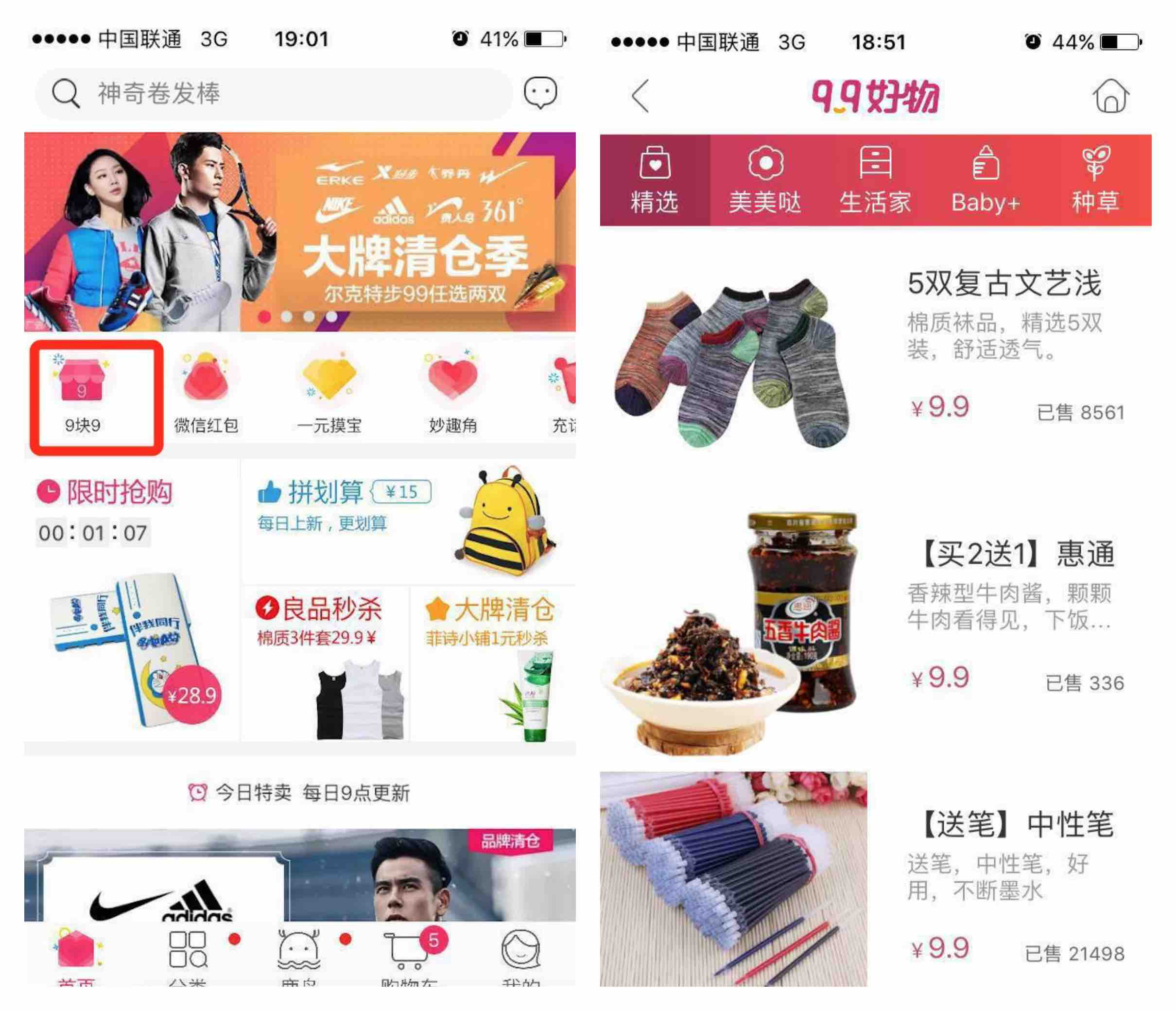### 问题背景### 分析问题

1. 如何定义用户对商品感兴趣？
2. 如何量化用户对商品的感兴趣程度，即如何对商品进行排序？
3. 如何解决用户冷启动？
4. 如何处理用户对9块9模块出现的新商品兴趣？

• 浏览（PV）
• 点击（CLICK）
• 收藏（FAV）
• 加购（CART）
• 下单（ORDER）

#### 量化感兴趣程度

$$态度 \in \{ 感兴趣，不感兴趣 \}$$

$$C \in \{C_{0}, C_{1}\}$$

$$\begin{eqnarray} P(C_{0}|U, I) \end{eqnarray}$$

### 商品排序

#### 朴素贝叶斯分离器

$U: (U_{0}, U_{1}, \dots, U_{m}).$

$I: (I_{1}, I_{1}, \dots, I_{n}).$

$$\begin{eqnarray} P(C_{0}|U_{0}, U_{1}, \dots, U_{m}, I_{1}, I_{1}, \dots, I_{n}) \end{eqnarray}$$

$$\begin{eqnarray} P(C_{0}|U, I) & = & P(C_{0}|U_{0}, U_{1}, \dots, U_{m}, I_{1}, I_{1}, \dots, I_{n}) \\ {} & = & \frac{P(C_{0}) \cdot P(U_{0}, U_{1}, \dots, U_{m}, I_{1}, I_{1}, \dots, I_{n}|C_{0})}{P(U_{0}, U_{1}, \dots, U_{m}, I_{1}, I_{1}, \dots, I_{n})} \\ {} & \approx & P(C_{0}) \cdot P(U_{0}, U_{1}, \dots, U_{m}, I_{1}, I_{1}, \dots, I_{n}|C_{0}) \\ {} & = & P(C_{0}) \cdot \prod_{i=1}^{m}P(U_{i}|C_{0}) \cdot \prod_{j=1}^{n}P(I_{j}|C_{0}) \end{eqnarray}$$

#### 统计特征概率计算

##### 计算 $P(C_{0})$

$P(C_{0})$ 表示用户对 9块9 模块商品的感兴趣概率。用户在逛9块9模块时，对列表中的商品会有5种行为：PV、CLICK、FAV、CART或ORDER。

$$P(C_{0}^{click}) = \frac{t_{2}}{t_{1}}$$

$$W = (w_{click}, w_{fav}, w_{cart}, w_{order}).$$

##### 计算 $P(U_{i}|C_{0})$

$P(U_{i}|C_{0})$ 表示具有特征 $U_{i}$ 的用户对 9块9 模块商品感兴趣概率。如果只考虑CLICK行为，并认为用户CLICK过商品，则表示用户对该商品感兴趣，假设9块9模块商品被点击的次数为 $t_{1}$，而具有特征 $U_{i}$ 的用户点击次数为 $t_{2}$，则

$$P(U_{i}|C_{0}^{click}) = \frac{t_{2}}{t_{1}}.$$

##### 计算 $P(I_{j}|C_{0})$

$P(I_{j}|C_{0})$ 表示具有特征 $I_{j}$ 的商品被用户感兴趣的概率。如果只考虑CLICK行为，并认为用户CLICK过商品，则表示用户对该商品感兴趣，假设9块9模块商品被点击的次数为 $t_{1}$，而具有特征 $I_{j}$ 的商品点击次数为 $t_{2}$，则

$$P(I_{j}|C_{0}^{click}) = \frac{t_{2}}{t_{1}}.$$

#### 用户对商品的感兴趣概率

$$\begin{eqnarray} P(C_{0}|U, I) & = & \sum_{b \in \{click, fav, cart, order\}}w_{b} \cdot P(C_{0}^{b}|U, I) \\ {} & \approx & \sum_{b \in \{click, fav, cart, order\}}w_{b} \cdot P(C_{0}^{b}) \cdot \prod_{i=1}^{m}P(U_{i}|C_{0}^{b}) \cdot \prod_{j=1}^{n}P(I_{j}|C_{0}^{b}) \end{eqnarray}$$